# Seeds

From a box of spruce seeds with the germination of 80%, we randomly select 10 seeds and plant them. Find the median of the random variable: the number of germinating seeds.

m =  8

### Step-by-step explanation: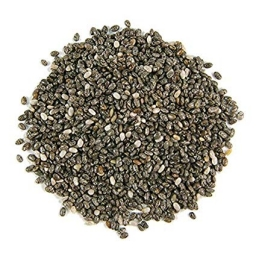Did you find an error or inaccuracy? Feel free to write us. Thank you!Tips to related online calculators
Looking for help with calculating arithmetic mean?
Looking for a statistical calculator?
Would you like to compute count of combinations?

#### You need to know the following knowledge to solve this word math problem:

We encourage you to watch this tutorial video on this math problem:

## Related math problems and questions:

• SeedsThe germination of seeds of a certain species of carrot is 96%. What is the probability that at least 25 seeds out of 30 will germinate?
• Population variance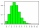In a California community college, 60% of students will transfer to a college in the CSU system. The number of students in a sample who will transfer follows a binomial distribution. If 8 students are randomly selected, find the population variance σ2 of
• SalesFrom statistics of sales goods, item A buy 51% of people and item B buys 59% of people. What is the probability that from 10 people buy 2 item A and 8 item B?
• FamilyWhat is the probability that a family with 7 childrens have: exactly 6 dívek? 2 dívky and 5 boys? Consider the birth probability of a girl is 48.87% and boy 51.13%.
• RecordsRecords indicate 90% error-free. If 8 records are randomly selected, what is the probability that at least 2 records have no errors?
• Statistics quizFill in the missing word 1. in a data set, the mean, median and mode are measured of ________________ 2. "The manipulation of variables under controlled conditions"is the data collection method known as______________ 3. in a normal distribution, the area
• Covid-19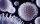Data showed that 22% of people in a small town was infected with the COVID-19 virus. A random sample of six residents from this town was selected. Find the probability that exactly two of these residents was infected.
• All use computerIt is reported that 72% of working women use computers at work. Choose 3 women at random, find the probability that all 3 women use a computer in their jobs.
• Class - boys and girls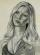In the class are 60% boys and 40% girls. Long hair has 10% boys and 80% girls. a) What is the probability that a randomly chosen person has long hair? b) The selected person has long hair. What is the probability that it is a girl?
• Seed germination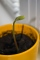Seed germination is 80%. 8% of the germinated plants subsequently die. How many seeds does a gardener have to sow in order to grow 2,000 plants?
• Family94 boys are born per 100 girls. Determine the probability that there are two boys in a randomly selected family with three children.
• Internet anywhere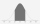In school, 60% of pupils have access to the internet at home. A group of 8 students is chosen at random. Find the probability that a) exactly 5 have access to the internet. b) At least 6 students have access to the internet
• Sample Proportion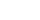In a California community college, 60% of students will transfer to a college in the CSU system. The number of students in a sample who will transfer follows a binomial distribution. 400 students are randomly selected from the college and 224 of them will
• Alopecia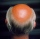Medical literature indicates that 45% of men suffer from alopecia. For random sample of 8 men, calculate the probability that: (a) exactly four men suffer from alopecia. (b) at most two men suffer from alopecia.
• Doctors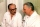The drug successfully treats 90% of cases. Calculate the probability that he will cure at least 18 patients out of 20?
• DiceWe throw 10 times a playing dice. What is the probability that the six will fall exactly 4 times?
• DiceWe throw five times the dice. What is the probability that six fits exactly twice?